Courses

# Chapter 3 Thyristor Commutation - Notes, Power Electronics, Electrical Engineering Electrical Engineering (EE) Notes | EduRev

## Electrical Engineering (EE) : Chapter 3 Thyristor Commutation - Notes, Power Electronics, Electrical Engineering Electrical Engineering (EE) Notes | EduRev

The document Chapter 3 Thyristor Commutation - Notes, Power Electronics, Electrical Engineering Electrical Engineering (EE) Notes | EduRev is a part of the Electrical Engineering (EE) Course Electrical Engineering SSC JE (Technical).
All you need of Electrical Engineering (EE) at this link: Electrical Engineering (EE)

Thyristor Commutation

The process of turning off the thyristor is termed as commutation. Special circuitry is employed for commutation of thyristor. A conducting thyristor can be turned off by natural or forced commutation.

1. Natural Commutation :

The thyristor current is reduced to zero due to the natural characteristics of the input voltage. The device is turned off due to natural behaviour of the source voltage with AC input voltage. Thyristor current goes through a natural zero and a reverse voltage appears across thyristor, hence, thyristor is turned off automatically due to natural behaviour of source voltage.
This method is used in
1. A.C. voltage controllers
2. Phase controlled rectifiers
3. Cycloconverters.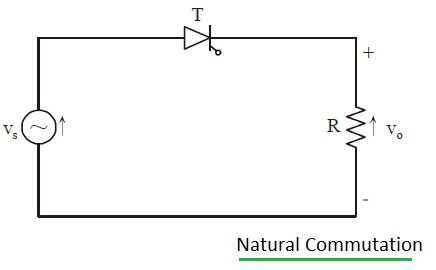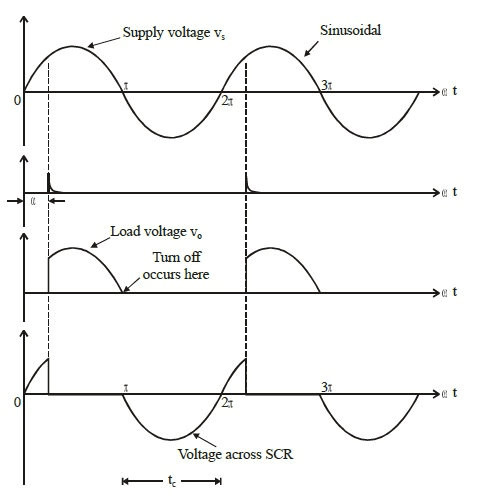2. Forced Commutation :

The thyristor current is reduced to zero by an additional circuit called a commutation circuit and turn off process depends on the load current.

Various types of forced commutation are
1. Self commutation
2. Complementary pulse commutation
3. Resonant commutation
4. Auxiliary commutation
5. Line commutation

(1) Self Commutation :

The circuit arrangement is as shown in figure.
When the thyristor is triggered, the capacitor is charged to a voltage higher than the supply voltage. At this instant, the current in the circuit is forced to turn-off the thyristor as the direction of current is reversed.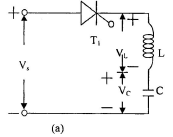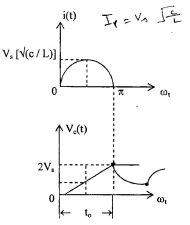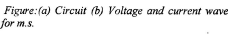(2) Complementry Pulse Commutation :

An auxiliary thyristor is used to turn off the main thyristor. The circuit is shown in figure. When Tis fired current flows through R2. At the same time, C is charged by CR1 combination. When Tis fired and conducts C discharges making cathode positive and anode negative. In this case the circuit is completed via R1 and capacitor gets charged through CR2 combination with the polarity opposite to the previous case. This cycle repeats alternately.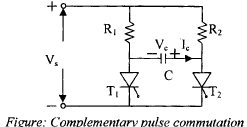(3) Resonant Commutation :

A parallel resonant circuit is used in the circuit, as shown in figure. The capacitor is charged initially with the polarities as shown. As the thyristor is turned on, two currents flow, a load current through the external circuit and pulse of current through LC circuit in the opposite direction. As the current through LC circuit (the discharge current) equals load current, thvristor turns-off.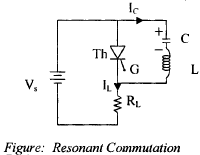(4) Auxiliary Commutation :

An auxiliary thyristor is used to turn-off the main thyristor, as shown in figure. Auxiliary thyristor ThA is triggered first so that the capacitor is charged with the polarities as shown. When C is fully charged to Vs ThA is turned-off naturally. When the main thyristor Thm is triggered the capacitor discharges through D, and its polarities reverse. Discharge of capacitor in reverse direction is prevented by D and commutation is achieved.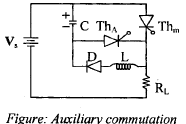(5) Line Commutation :

In case of AC supply to the thyristor voltage is applied to it during the negative half cycle. If the duration of the negative cycle is longer than the turn- off time, the thyristor is turned off.

Offer running on EduRev: Apply code STAYHOME200 to get INR 200 off on our premium plan EduRev Infinity!

65 docs|37 tests

,

,

,

,

,

,

,

,

,

,

,

,

,

,

,

,

,

,

,

,

,

,

,

,

,

,

,

;